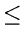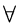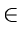Next: 4.8.3 Splay Trees Up: 4.8 Problems Previous: 4.8.1 General Trees

## 4.8.2 Binary Search Trees

1.
Suppose that we have numbers between 1 and 1000 in a binary search tree and want to search for the number 363. Which of the following sequences could not be the sequence of nodes examined?
(a)
2, 252, 401, 398, 330, 344, 397, 363.
(b)
924, 220, 911, 244, 898, 258, 362, 363.
(c)
925, 202, 911, 240, 912, 245, 363.
(d)
2, 399, 387, 219, 266, 382, 381, 278, 363.
(e)
935, 278, 347, 621, 299, 392, 358, 363.
2.
In a binary search tree, given a key x, define successor(x) as the key which is the successor of x in the sorted order determined by an inorder tree walk. Define predecessor(x) similarly. Explain how, given x, its successor and predecessor may be found.
3.
A binary search tree is constructed by inserting the key values 1, 2, 3, 4, 5, 6, 7 in some order specified by a permutation of 1, ..., 7, into an initially empty tree. Which of these permutations will lead to a complete binary search tree ( 1 node at level 1, 2 nodes at level 2, and 4 nodes at level 3)?
4.
Suppose that the search for key k in a binary search tree ends up in a leaf. Consider three sets: A, the keys to the left of the search path; B, the keys on the search path; and C, the keys to the right of the search path. Investigate if the following is true: abcaA; bB; cC. Give a proof or a counter-example as the case may be.
5.
Is the operation of deletion in a binary search tree commutative in the sense that deleting x and then y from a binary search tree leaves the same tree as deleting y and then x? Argue why it is so or give a counter-example.

6.
Devise an algorithm that takes two values, a and b such that ab, and visits all keys x in a BST such that axb. The running time of your algorithm should be O(N + log n), where Nis the number of keys visited and n is the number of keys in the tree.

7.
A random binary search tree having n nodes, where n = 2k - 1, for some positive integer k, is to be reorganized into a perfectly balanced binary search tree. Outline an efficient algorithm for this task. The algorithm should not use any memory locations other than those already used by the random binary search tree.

8.
Consider three keys, k1, k2, k3, such that k1 < k2 < k3. A binary search tree is constructed with these three keys. Depending on the order in which the keys are inserted, five different binary search trees are possible.
(a)
Write down the five binary search trees.
(b)
Let p, q, r be the probabilities of probing for k1, k2, k3, respectively in the given binary search trees (p + q + r = 1). Compute the average number of comparisons (or probes) on a successful search for each of the above five binary search trees.
(c)
Define the optimum binary search tree as the one for which the average number of comparisons on a successful search is minimum. Determine the range of values of p, q, r, for which the completely balanced search tree is the optimum search tree.
9.
Given a set of 31 names, each containing 10 uppercase alphabets, we wish to set up a data structure that would lead to efficient average case performance of successful and unsuccessful searches on this set. It is known that not more than 5 names start with the same alphabet. Also, assume that successful and unsuccessful searches are equally likely and that in the event of a successful search, it is equally likely that any of the 31 names was searched for. The two likely choices for the data structure are:
• A closed hash table with 130 locations where each location can accommodate one name.
• A full binary search tree of height 4, where each of the 31 nodes contains a name and lexicographic ordering is used to set up the BST.